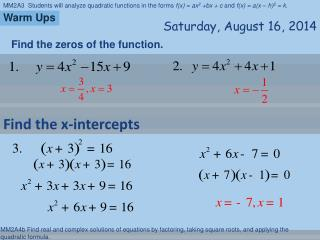# Warm Ups - PowerPoint PPT PresentationDownload PresentationWarm Ups

Warm UpsDownload Presentation## Warm Ups

- - - - - - - - - - - - - - - - - - - - - - - - - - - E N D - - - - - - - - - - - - - - - - - - - - - - - - - - -
##### Presentation Transcript

1. Warm Ups Saturday, August 16, 2014 Find the zeros of the function. Find the x-intercepts

2. Solving Quadratic Equation by Finding Square Roots Saturday, August 16, 2014 Essential Question: How do we use the square root property?

3. Vocabulary

4. Key Concept • Isolate the radical. • Take the square root. • Simplify the radical.

5. Example Solve the quadratic equation.

6. Guided Practice Solve the quadratic equation.

7. Guided Practice Solve the quadratic equation.

8. Guided Practice Solve the quadratic equation.

9. Guided Practice Solve the equation.

10. Falling Objects! Key Concept Use h = -16t2 + h0 Height of the object after it has fallen Object’s initial height # of seconds after the object is dropped

11. Example 6. The tallest building in the USA is in Chicago, Illinois. It is 1450 ft. tall. How long would it take a penny to drop from the top of the building to the ground?

12. 7. A rescue helicopter hovers 68 feet above a jet ski in distress and drops a life raft. The height in feet of the raft above the water is given byh(t) = -16t2 + 68. Determine how long it will take for the raft to hit the water after being dropped from the helicopter. Solution: The raft will hit the water when its height is 0 feet above the water. Solve h(t) = -16t2 + 68 = 0. -16t2 + 68 = 0 Ignore the - 2.1. WHY? -16t2 = - 68 The life raft will hit about 2.1 seconds after it is dropped.

13. For a science competition, students must design a container that prevents an egg from breaking when dropped from a height of 50 feet. How long does the container take to hit the ground? = t2 5016 50 = t2 + 16 Model a dropped object with a quadratic function 8. Science Competition Use h = – 16t 2 + 50 0 = – 16t 2 + 50 + 1.8 ≈t – 50 = – 16t 2 Reject the negative solution, – 1.8, because time must be positive. The container will fall for about 1.8 seconds before it hits the ground.